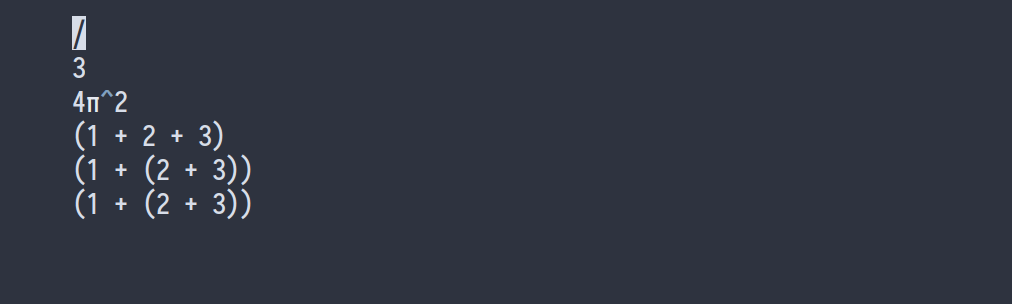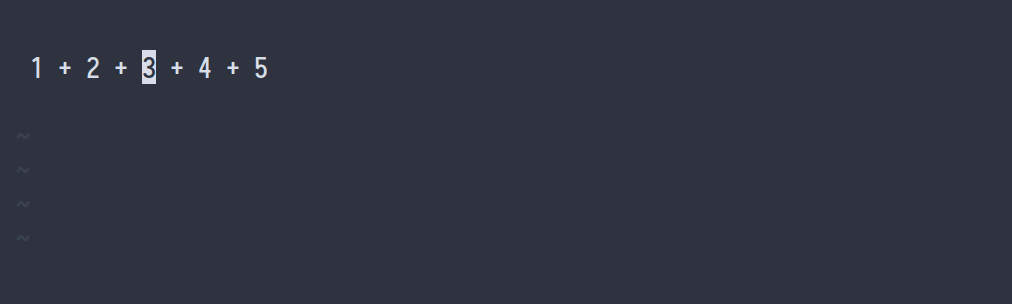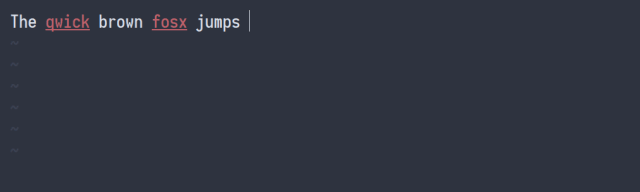# 世界上最好的编辑器Vim：1700多页数学笔记是如何实时完成的图片截图来自 CS224n 2019• 利用 LaTex 记文本和数学公式应与讲师在黑板上写字的速度保持一致：不允许延误。

• 画图的速度应尽量与讲师保持一致。

• 做笔记，如添加注解、编辑所有注解、整合最后两堂课的内容、搜索注解等，应方便快捷。

• 当我们想在 pdf 文件旁边添加注释时，利用 LaTex 应能够实现这一目的。

Vim 和 LaTexPlug 'lervag/vimtex'
let g:tex_flavor='latex'
let g:vimtex_view_method='zathura'
let g:vimtex_quickfix_mode=0
set conceallevel=1
let g:tex_conceal='abdmg'


!p snip.rv = '└' + '─' * (len(t) + 2) + '┘'
$0 endsnippet  这些 Python 代码块将会被变量 snip.rv 的值替代。在这些代码块内部，你可以访问 snippet 的当前状态，如 t 包含第一个制表位，fn 表示当前文档名称。 LaTeX snippet 借助于 snippet，利用 LaTeX 做笔记要比手写快得多。一些复杂的 snippet 可以为你节省大量时间并消除做笔记的挫败感。让我们开始了解一些简单的 snippet。 环境 为了嵌入环境，我必须在一行开端键入 beg。之后我键入环境名称，后者会直接在\end{} 指令中映出。按下 Tab 使光标位于新创建的环境中。该 snippet 的代码如下所示： snippet beg "begin{} / end{}" bA \begin{$1}
$0 \end{$1}
endsnippet


b 意味着该 snippet 将只能在一行开端扩展，并且 A 代表自动扩展，这意味着我不需要键入 Tab 就可以扩展 snippet。制表位--即可以通过按下 Tab 和 Shift+Tab 跳转到的地方--以$1、$2 等表示，同时最后一个为$0。 inline math 和 display math 我最常使用的两个 snippet 是 mk 和 dm。这些 snippet 负责数学代码的开始。第一个是 inline math snippet，第二个是 display math snippet。in­line math snippet 是「智能的」：它知道何时在$符号后嵌入一个位置。当我在结尾$的正后方开始键入一个单词时，它添加一个位置。但是，当我键入一个非单词字符时，它不添加一个位置，例如下图的$p$-value。该 snippet 的代码如下所示： snippet mk "Math" wA$${1}$!pif t and t not in [',', '.', '?', '-', ' ']:
snip.rv = ' 'else:
snip.rv = ''
$2endsnippet  第一行结尾处的 w 意味着该 snippet 将在词边界扩展，所以举例而言，hellomk 不会扩展，而 hello mk 会扩展。 diaplay math snippet 更简单，但同时也相当方便；该 snippet 使我在一段时间内不会忘记结束方程式。snippet dm "Math" wA $1 .$$0
endsnippetsnippet '([A-Za-z])(\d)' "auto subscript" wrA
!p snip.rv = match.group(1)_!p snip.rv = match.group(2)
endsnippet

snippet '([A-Za-z])_(\d\d)' "auto subscript2" wrA
!p snip.rv = match.group(1)_{!p snip.rv = match.group(2)}
endsnippetsnippet sr "^2" iA
^2
endsnippet

snippet cb "^3" iA
^3
endsnippet

snippet compl "complement" iA
^{c}
endsnippet

snippet td "superscript" iA
^{$1}$0
endsnippetsnippet // "Fraction" iA
\\frac{$1}{$2}$0 endsnippet  第二和第三个例子使用正则表达式匹配 3/、4ac、 6\pi^2/、a_2/等表达式。 snippet '((\d+)|(\d*)(\\)?([A-Za-z]+)((\^|_)(\{\d+\}|\d))*)/' "Fraction" wrA \\frac{!p snip.rv = match.group(1)}{$1}$0 endsnippet  如你所见，正则表达式可能非常复杂，这里有个图表可以解释：在第四、第五个例子中，它试图找到匹配圆括弧。由于不能使用 Ul­tiSnips 的正则表达式引擎，我转向了 Python： priority 1000 snippet '^.*\)/' "() Fraction" wrA !p stripped = match.string[:-1] depth = 0 i = len(stripped) - 1 while True: if stripped[i] == ')': depth += 1 if stripped[i] == '(': depth -= 1 if depth == 0: break; i -= 1 snip.rv = stripped[0:i] + "\\frac{" + stripped[i+1:-1] + "}" {$1}$0 endsnippet  最后一个与分数有关的 snippet 即使用你的选择来制作分数。你可以先用它选择一些文本，然后按下 Tab，打出/，然后再按下 Tab。这些代码用到了${VISUAL} 变量表示你的选择。

snippet / "Fraction" iA
\\frac{${VISUAL}}{$1}$0 endsnippet  sympy 和 Math­e­mat­i­ca 另一个很酷但不太常用的 snippet 是利用 sympy 评估数学表达式。例如，sympy Tab 键扩展为 sympy | sympy，sympy 1 + 1 sympy Tab 键扩展为 2。snippet sympy "sympy block " w sympy$1 sympy$0 endsnippet priority 10000 snippet 'sympy(.*)sympy' "evaluate sympy" wr !p from sympy import * x, y, z, t = symbols('x y z t') k, m, n = symbols('k m n', integer=True) f, g, h = symbols('f g h', cls=Function) init_printing() snip.rv = eval('latex(' + match.group(1).replace('\\', '') \ .replace('^', '**') \ .replace('{', '(') \ .replace('}', ')') + ')')  endsnippet  Math­e­mat­i­ca 用户也可以进行类似的操作：priority 1000 snippet math "mathematica block" w math$1 math$0 endsnippet priority 10000 snippet 'math(.*)math' "evaluate mathematica" wr !p import subprocess code = 'ToString[' + match.group(1) + ', TeXForm]' snip.rv = subprocess.check_output(['wolframscript', '-code', code])  endsnippet  后缀 snippet 值得分享的还有后缀 snippet，如 phat → \hat{p}，zbar → \overline{z} 等。一个类似的 snippet 是后缀向量，如 v → \vec{v}。这些 snippet 真的非常节省时间，因为你可以借此跟上老师板书的节奏。注意，我还是可以用 bar 和 hat 前缀，我以较低的优先级将它们添了进去。那些 snippet 的代码如下： priority 10 snippet "bar" "bar" riA \overline{$1}$0 endsnippet priority 100 snippet "([a-zA-Z])bar" "bar" riA \overline{!p snip.rv=match.group(1)} endsnippet priority 10 snippet "hat" "hat" riA \hat{$1}\$0
endsnippet

priority 100
snippet "([a-zA-Z])hat" "hat" riA
\hat{!p snip.rv=match.group(1)}
endsnippet

snippet "(\\?\w+)(,\.|\.,)" "Vector postfix" riA
\vec{!p snip.rv=match.group(1)}
endsnippetglobal !p
texMathZones = ['texMathZone'+x for x in ['A', 'AS', 'B', 'BS', 'C',
'CS', 'D', 'DS', 'E', 'ES', 'F', 'FS', 'G', 'GS', 'H', 'HS', 'I', 'IS',
'J', 'JS', 'K', 'KS', 'L', 'LS', 'DS', 'V', 'W', 'X', 'Y', 'Z']]

texIgnoreMathZones = ['texMathText']

texMathZoneIds = vim.eval('map('+str(texMathZones)+", 'hlID(v:val)')")
texIgnoreMathZoneIds = vim.eval('map('+str(texIgnoreMathZones)+", 'hlID(v:val)')")

ignore = texIgnoreMathZoneIds

def math():
synstackids = vim.eval("synstack(line('.'), col('.') - (col('.')>=2 ? 1 : 0))")
try:
first = next(
i for i in reversed(synstackids)
if i in texIgnoreMathZoneIds or i in texMathZoneIds
)
return first != ignore
except StopIteration:
return False
endglobalsetlocal spell
set spelllang=nl,en_gb
inoremap <C-l> <c-g>u<Esc>[s1z=]a<c-g>u